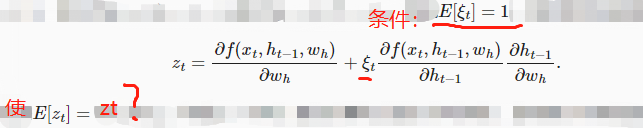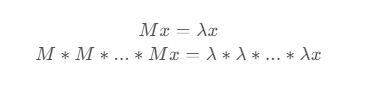# 通过时间反向传播

@goldpiggy Hey, I can help do some translations.

1 Like

come on！
1 Like

5 Likes
1. 注意：这里文章里讲的隐藏层中的一个权重变量Wh指的是[[Wxh],[Whh]] 按axis=0拼接。

2. 请问，这是怎么推导出来的？3. 为什么第一个式子进行求和，第二个式子没有呢？

2 Likes

2:：根据数学期望的性质E[AX+B] = B+AE[X]很容易计算出来
3：T是最后一个时间步。对于hT的计算图为L ← oT <—hT * WT, 因此不需要累加

2 Likes

1 Like

1 Like

$$M^k v_i = M^(k-1) M v_i = M^(k-1) \lambda_i v_i = … = \lambda_i^k v_i$$

$$x = sum( a_i v_i, from i = 1 to n)$$

$$M^k x = M^k sum( a_i v_i, from i = 1 to n)$$
$$= sum( a_i M^k v_i, from i = 1 to n)$$
$$= sum( a_i \lambda_i^k v_i, from i = 1 to n)$$